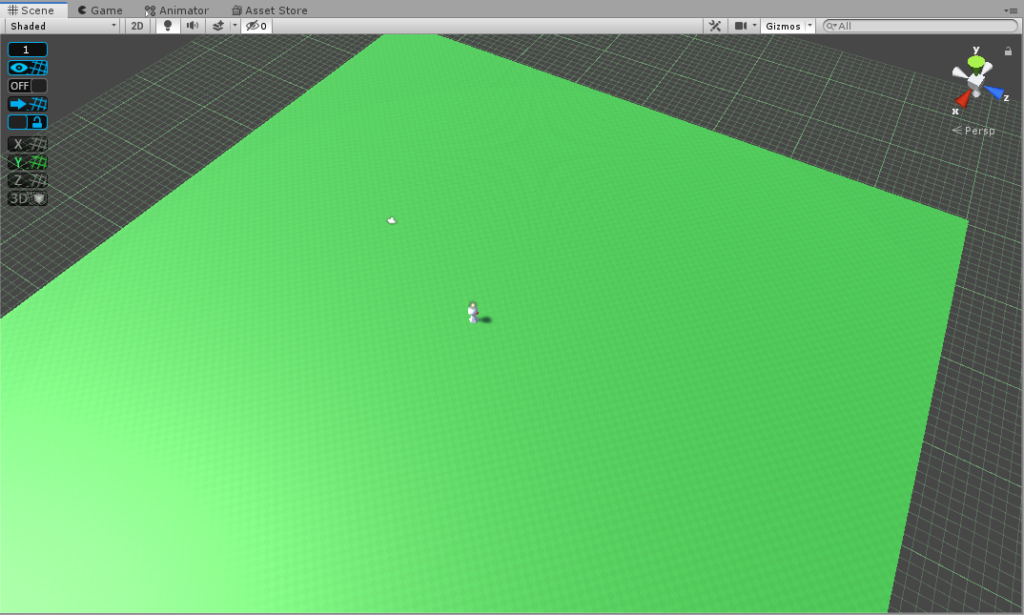Hi everynyan, Saishy here!

So I’ve decided to try meddling a little in 3D dev using Unity3D and I will be posting anything I find interesting along.

First I’ve got some packages, ProBuilder, ProGrids, PolyBrush, ShaderGraph… Then I did some 10 per 10 tiles and cloned them to get a long enough play field:The player character is a little placeholder I made with a sphere, cone and a cylinder, I also added some animations to give it personality!

I’ve had to decide on how I want my movement, there is actually a lot of ways, it’s horrible!

• Transform: You have to code everything, don’t have physics by itself.
• CharacterController: You have to code all kinds of movement, can collide by itself.
• Velocity: You have to deal with any physics interaction by yourself, multiple forces, attrition etc. (May have unpredictable moments)
• Force: Janky, less feeling of control. (Same unpredictable moments problem).

And some minor things like kinematic/non-kinematic and others.

I’ve ended up using CharacterController, because it reminds me of Unreal movement and I liked that.
To control it I separated gathering the input into a Controller script and receiving that input in a Pawn script:

```// FixedUpdate is called once per physics frame
void FixedUpdate() {
Vector3 speed = Vector3.zero;

if (_movementInput.sqrMagnitude > 0) {
speed = _movementInput * MovementSpeed;

_charController.SimpleMove(speed);

transform.rotation = Quaternion.LookRotation((transform.position + _movementInput) - (transform.position));
//transform.rotation = Quaternion.RotateTowards(transform.rotation, Quaternion.LookRotation((transform.position + _movementInput) - (transform.position)), 4f);
}

_animator.SetInteger("SpeedXZ", Mathf.Abs((int)speed.sqrMagnitude));
_animator.SetInteger("SpeedX", Mathf.Abs((int)(speed.x * 10)));
_animator.SetInteger("SpeedZ", Mathf.Abs((int)(speed.z * 10)));
}```
```// Update is called once per frame
void Update() {
Vector2 movementInput = Vector2.zero;

movementInput.x = player.GetAxis("Move Horizontal");
movementInput.y = player.GetAxis("Move Vertical");

}```

I’m using the Rewired asset for getting the input, it’s a great asset!

And… Oops! I forgot to normalize the movement so you can walk faster diagonally! I was in doubt if I should normalize the input before sending or after receiving but after seeing that joysticks weren’t able to reach (1, 1) in Rewired I choose for before the input so both keyboard and joysticks are the same:

```// Update is called once per frame
void Update() {
Vector2 movementInput = Vector2.zero;

movementInput.x = player.GetAxis("Move Horizontal");
movementInput.y = player.GetAxis("Move Vertical");

movementInput.Normalize();

}```

Now I want some jumps! This will actually require an overhaul of everything:

```// Update is called once per frame
void Update() {
Vector2 movementInput = Vector2.zero;

movementInput.x = player.GetAxis("Move Horizontal");
movementInput.y = player.GetAxis("Move Vertical");

movementInput.Normalize();

_playerPawn.Jump(player.GetButton("Jump"));
}```
```protected Vector3 _movementInput;
protected float _jumpSpeed;
protected float _gravity;

// FixedUpdate is called once per physics frame
void FixedUpdate() {
Vector3 speed = Vector3.zero;

if (_charController.isGrounded) {
_gravity = Physics.gravity.y * Time.fixedDeltaTime;
}

if (_movementInput.sqrMagnitude > 0) {
speed += (_movementInput * MovementSpeed);
//transform.rotation = Quaternion.RotateTowards(transform.rotation, Quaternion.LookRotation((transform.position + _movementInput) - (transform.position)), 4f);
}

speed.y += _jumpSpeed + _gravity;

_charController.Move(speed * Time.fixedDeltaTime);

if (_movementInput.sqrMagnitude > 0) {
transform.rotation = Quaternion.LookRotation((transform.position + _movementInput) - (transform.position));
}

// Remember to multiply gravity by time twice since gravity is an acceleration.
_jumpSpeed += Physics.gravity.y * Time.fixedDeltaTime;
if (_jumpSpeed < 0) {
_jumpSpeed = 0;
}

_gravity += Physics.gravity.y * Time.fixedDeltaTime;

Vector3 velocity = _charController.velocity;
velocity.y = 0;

_animator.SetInteger("SpeedXZ", Mathf.Abs((int)velocity.sqrMagnitude));

velocity.z = 0;
_animator.SetInteger("SpeedX", Mathf.Abs((int)(velocity.sqrMagnitude)));

velocity.x = 0;
velocity.y = _charController.velocity.y;
_animator.SetInteger("SpeedY", Mathf.Abs((int)(velocity.sqrMagnitude)));

velocity.y = 0;
velocity.z = _charController.velocity.z;
_animator.SetInteger("SpeedZ", Mathf.Abs((int)(velocity.sqrMagnitude)));
}

_movementInput.x = input.x;
_movementInput.z = input.y;
}

public void Jump(bool bJump) {
if (_charController.isGrounded && bJump) {
_jumpSpeed = 1f * JumpSpeed;
}
}```

And the result is:

Cute isn’t it??!

Oh no I forgot about camera following!

```using System.Collections;
using System.Collections.Generic;
using UnityEngine;

namespace CraftTest {

public class CameraController : MonoBehaviour {

public static CameraController instance;

//Distance to character
private Vector3 _offset;

void Awake() {
_offset = transform.localPosition;

instance = this;
}

public void LookAt(Vector3 position) {
transform.localPosition = position + _offset;
}
}
}```

Now just add CameraController.instance.LookAt(_playerPawn.transform.position); to the end of the Controller script and we are done:

This will be all for today’s post, thanks for reading!

This site uses Akismet to reduce spam. Learn how your comment data is processed.